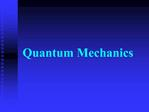DownloadDownload PresentationQuantum Mechanics

# Quantum Mechanics

Télécharger la présentation## Quantum Mechanics

- - - - - - - - - - - - - - - - - - - - - - - - - - - E N D - - - - - - - - - - - - - - - - - - - - - - - - - - -
##### Presentation Transcript

1. Quantum Mechanics

2. The Bohr Model Electrons travel in discrete NRG levels around the nucleus. When they absorb NRG the �jump� to a higher level�an excited state. In order to return to ground state this NRG must be released in the form of a photon. The NRG released or absorbed can be calculated.

3. Calculating NRG E = -Rh (1/n2) Rh = 2.18 x 10-18J ?E = NRG of Final � NRG of Initial Works well only for hydrogen atoms. The quantization of energy is right, but electrons are not circling the nucleus.

4. The Quantum Mechanical Model A totally new approach. De Broglie said matter could be like a wave. De Broglie said they were like standing waves. ie: the vibrations of a stringed instrument.

6. What�s possible? You can only have a standing wave if you have complete waves. There are only certain allowed waves. In the atom there are certain allowed waves called electrons. 1925 Erwin Schr�edinger described the wave function of the electron. Way too much math, but what is important is the solution.

7. Schr�edinger�s Equation The wave function represents a coordinate (x,y,and z) where an electron may reside. Solutions to the equation are called orbitals. (not Bohr orbits) Each solution is tied to a certain energy. These are the energy levels.

8. The Heisenberg Uncertainty Principle There is a limit to what we can know. There is a limitation to how well we can know both the position and the momentum of an object. The more precisely we know the position, the less accurately we know the momentum.

9. Mathematically Dx � D(mv) ? h/4p Dx is the uncertainty in the position. D(mv) is the uncertainty in the momentum. the minimum uncertainty is h/4p

10. Given these limitations, then what does the wave Function mean? nothing. It is not possible to visually map it. But�.The square of the function is the probability of finding an electron near a particular spot. best way to visualize it is by mapping the places where the electron is most likely to be found.

11. Defining the size of the orbital: Electron density map The size that encloses 90% to the total electron probability. NOT at a certain distance, but a most likely distance to find the e-. For the first solution it is a sphere.

12. Quantum Numbers There are many solutions to Schroedinger�s equation. Each solution can be described with quantum numbers that describe some aspect of the solution. There are four quantum numbers that describe the probable location of the electron.

13. Principle Quantum Number (n) Determines the size and energy of of an orbital. Has integer values >0 Higher �n� value = Higher NRG

14. Angular momentum quantum number ( l ). Defines the shape of the orbital. integer values from 0 to n-1 l = 0 is called s l = 1 is called p l =2 is called d l =3 is called f l =4 is called g

21. Magnetic quantum number (m l) integer values between - l and + l tells direction in each shape. For S: l=0 therefore ml = 0 For P: l=1 �. ml = -1, 0, or +1 For D: l=2 �ml = -2, -1, 0, +1, +2 For F:��� you get it�..

22. Electron spin quantum number (m s) Electrons in the same orbital have opposite spins. Can have 2 values. either +1/2 or -1/2

23. Practice: Which set is not allowed? n=1, l=0, ml=1 n=2, l=2, ml=1 n=5, l=3, ml=2 n=6, l=-2, ml=2 n=6, l=2, ml=-2

24. Practice: Which set is not allowed? n=3, l=3, ml=0, ms = -� n=4, l=3, ml=2, ms = -� n=4, l=1, ml=1, ms = +� n=2, l=1, ml=-1, ms = -1 n=5, l=-4, ml=2, ms = +�